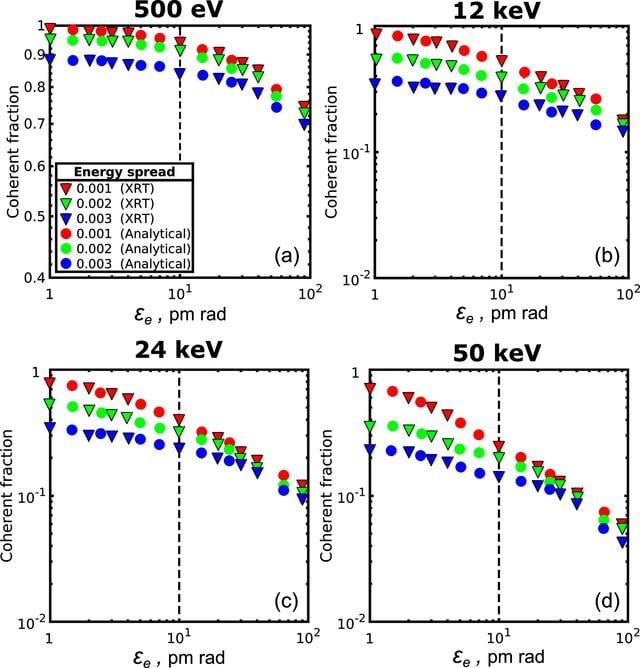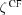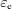disable zoom     view article Figure 5 Coherent fraction of radiationas a function of the electron beam emittancefor the different values of the photon energy and energy spread in one transverse direction. Triangles are XRT simulations, circles are analytical calculations performed according to equation (13). Red, green and blue colour correspond to 0, 1 × 10−3 and 2 × 10−3 relative energy spread values, respectively. Dashed vertical line corresponds to the value of the electron emittance of 10 pm rad. Note the different scale for 500 eV coherent fraction value.JOURNAL OFSYNCHROTRONRADIATION
ISSN: 1600-5775
Volume 26| Part 6| November 2019| Pages 1851-1862